Open In App
Related Articles
• RD Sharma Class 9 Solutions

# Class 9 RD Sharma Solutions – Chapter 8 Introduction to Lines and Angles- Exercise 8.2 | Set 1

### Question 1: In the below Fig. OA and OB are opposite rays:

(i) If x = 25°, what is the value of y?

(ii) If y = 35°, what is the value of x?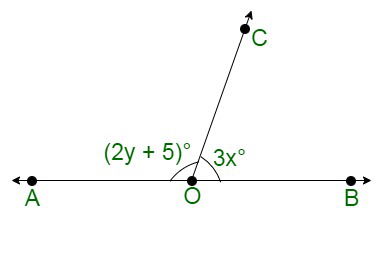Solution:

(i) Given:

x = 25

In the figure;

∠AOC and ∠BOC are forming a linear pair

So, ∠AOC + ∠BOC = 180°

In the figure;

∠AOC = 2y + 5 and ∠BOC = 3x

∠AOC + ∠BOC = 180°

(2y + 5) + 3x = 180

(2y + 5) + 3 (25) = 180

2y + 5 + 75 = 180

2y + 80 = 180

2y = 100

y == 50

Hence,

y = 50°

(ii) Given:

y = 35°

In the figure;

∠AOC + ∠BOC = 180° {Linear pair angles}

(2y + 5) + 3x = 180

(2(35) + 5) + 3x = 180

75 + 3x = 180

3x = 105

x = 35

Therefore, x = 35°

### Question 2. In the below figure, write all pairs of adjacent angles and all the linear pairs.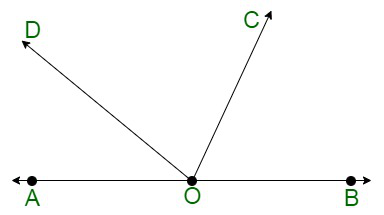Solution

In the figure;

(∠AOC, ∠COB);

(∠AOD, ∠BOD);

(∠AOD, ∠COD);

(∠BOC, ∠COD)

∠AOD + ∠BOD = 180° {Linear pair}

and

∠AOC+ ∠BOC = 180° {Linear pair}

### Question 3. In the given figure, find x. Further find ∠BOC, ∠COD, and ∠AOD.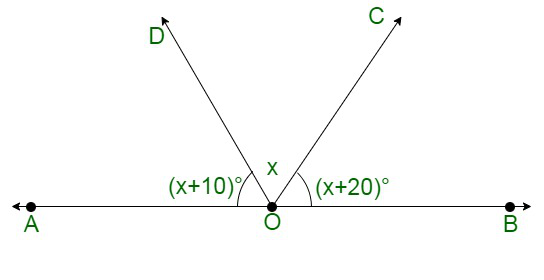Solution:

In the figure;

∠AOD and ∠BOD are forming linear pair,

Thus,

∠AOD+ ∠BOD = 180°

And,

∠AOD + ∠BOC + ∠COD = 180°

Given:

∠AOD = (x+10)°,

∠COD = x°

and

∠BOC = (x + 20)°

(x + 10) + x + (x + 20) = 180°

3x + 30 = 180°

3x = 180 – 30

x =x = 50°

Here,

∠AOD = (x+10) = 50 + 10 = 60

∠COD = x = 50°

∠BOC = (x+20) = 50 + 20 = 70°

Therefore,

∠AOD = 60°,

∠COD = 50°

and

∠BOC=70°

### Question 4. In figure, rays OA, OB, OC, OD, and OE have the common endpoint 0. Show that ∠AOB+∠BOC+∠COD+∠DOE+∠EOA=360°.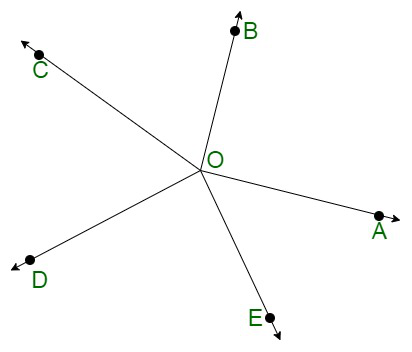Solution:

Given:

Rays OA, OB, OC, OD and OE have the common endpoint O.

Construct: Draw an opposite ray OX to ray OA, which make a straight line AX.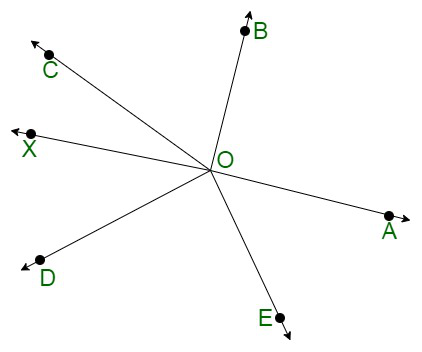In the figure:

∠AOB + ∠BOX = 180° {Linear pair}

Or,

∠AOB + ∠BOC + ∠COX = 180° ……….(i)

Also,

∠AOE + ∠EOX  = 180°  {Linear pair}

Or,

∠AOE + ∠DOE + ∠DOX = 180° ………..(ii)

After adding equations, (i) and (ii), we will get;

∠AOB + ∠BOC + ∠COX + ∠AOE + ∠DOE + ∠DOX = 180° + 180°

∠AOB + ∠BOC + ∠COD + ∠DOE + ∠EOA = 360°

Hence, Proved.

### Question 5. In figure, ∠AOC and ∠BOC form a linear pair. If a – 2b = 30°, find a and b?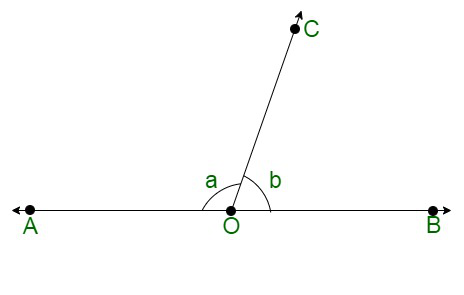Solution:

Given:

∠AOC and ∠BOC are forming a linear pair.

a + b = 180° …….(i)

a – 2b = 30° …….(ii) {given}

After subtracting equation (ii) from (i), we will get

a + b – a + 2b = 180 – 30

3b = 150

b =b = 50°

Thus,

a – 2b = 30°

a – 2(50) = 30°

a = 30 + 100

a = 130°

Hence,

a = 130°

b = 50°

### Question 6. How many pairs of adjacent angles are formed when two lines intersect at a point?

Solution

Here, the four pairs of adjacent angles are formed when two lines intersect each other at a single point.

So here Let two lines AB and CD intersect at point O as shown below in the figure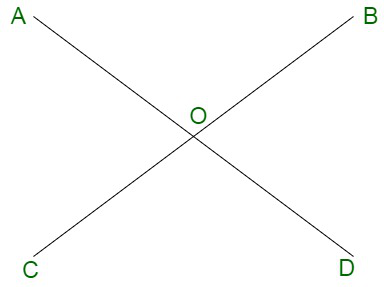Thus, the 4 pair of adjacent angles are :

(∠AOD, ∠DOB),

(∠DOB, ∠BOC),

(∠COA, ∠AOD)

and

(∠BOC, ∠COA)

### Question 7. How many pairs of adjacent angles, in all, can you name in the figure given?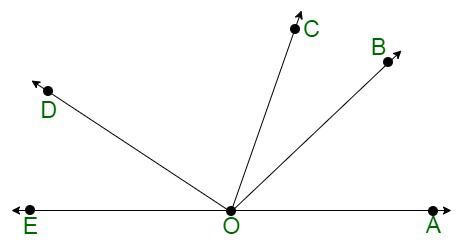Solution

The number of Pairs of adjacent angles, from the following figure are;

∠EOC and ∠DOC

∠EOD and ∠DOB

∠DOC and ∠COB

∠EOD and ∠DOA

∠DOC and ∠COA

∠BOC and ∠BOA

∠BOA and ∠BOD

∠BOA and ∠BOE

∠EOC and ∠COA

∠EOC and ∠COB

Thus, we have 10 pairs of adjacent angles.

### Question 8. In figure, determine the value of x.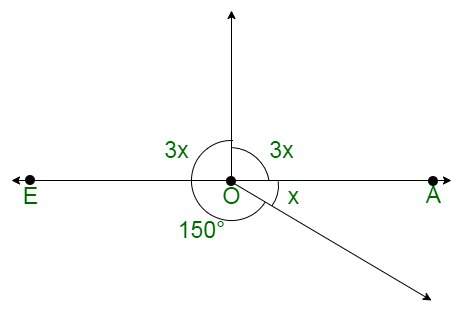Solution:

As we know that, the sum of all the angles around a point O is equal to 360°.

Thus,

3x + 3x + 150 + x = 360°

7x = 360° – 150°

7x = 210°

x =x = 30°

Hence, the value of x is 30°.

### Question 9. In figure, AOC is a line, find x.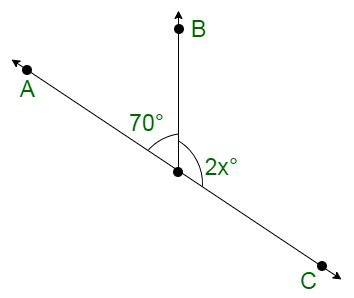Solution:

In the following figure,

∠AOB + ∠BOC = 180° {Linear pairs}

70 + 2x = 180

2x = 180 – 70

2x = 110

x =x = 55°

Hence, the value of x is 55°.

### Question 10. In figure, POS is a line, find x.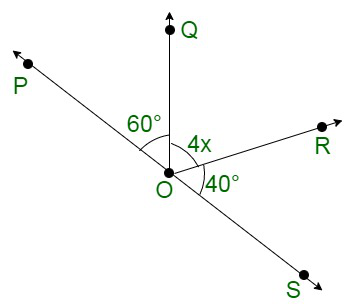Solution:

In the following figure;

∠POQ + ∠QOS = 180° {Linear pair}

∠POQ + ∠QOR +∠SOR = 180°

60° + 4x + 40° = 180°

4x = 180° -100°

4x = 80°

x = 20°

Thus, the value of x is 20°.